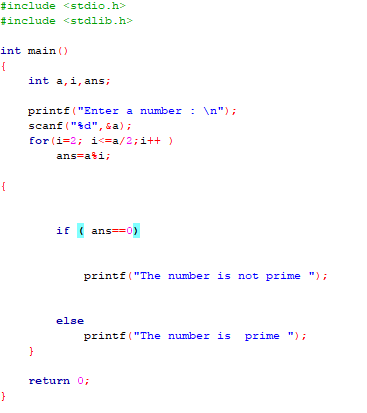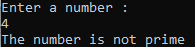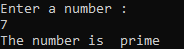# Concepts of electrical and electronics engineering

The book is written per the syllabus of first year engineering degree course for various universities. It covers basic topics of electrical, electronics and communication engineering. It also includes worked out examples, University examination questions and answers, exercise, etc in every chapter. This book is suitable for course in basic electrical engineering under various Universities.
Authors have tried to elucidate the topics in such a way that even a mediocre student can assimilate them. Many solved problems, sample question papers and exercise given in every section will provide a thorough understanding of the topics. Other features include attractive writing style, well structured equations and numerical examples, pictures of high clarity, etc.

# Applied Mathematics - 1 Book

• Introduction to Differentiation
• Differential Calculus
• Introduction to Integration
• Integral Calculus
• Ordinary Differential Equations
• Vector Calculus
• Tutorial Sheets
• Previous Years Examination Papers
• Index

# Lecture - 24 Case Study

# Lecture - 23 Case Study

# Lecture - 22 Verification and Validation

# Lecture - 21 Software Metrics and Quality

# Lecture - 20 Structural Programming and Some implementation

# Lecture - 19 Software Testing - II

# Lecture - 18 Software Testing - I

# Lecture - 16 Class and Component Level Design

# Lecture - 15 Design Patterns

# Lecture - 14 Software Design - Primary Consideration

# Lecture -13 Production Quality Software - Introduction

# Lecture-12 Data Modelling

# Lecture - 11 Data Modelling - ER Diagrams, Mapping

# lecture - 9 Process Modelling - DFD , Function Decomp

# Lecture - 10 Process Modelling - DFD, Function Decomp

# Lecture - 8 Systems Modelling Overview

# Lecture - 7 Algebraic Specification Methods

# Lecture - 6 Formal Specification

# Lecture -5 Requirements Engineering / Specification

# Lecture - 4 Overview of Phases

# Lecture-3 Overview of Phases

# Lecture - 2 Introduction to Software Engineering

# Lecture - 1 Introduction to Software Engineering

# Program in C for multiplication table using while loop

Input :-

Output :-

Code :-

#include <stdio.h>
int main()
{
int n,i=1;
printf("Enter a number :");
scanf("%d",&n);
while (i<=10)
{
printf("%d * %d = %d \n", n, i, n*i);
i++;
}
}

# Print multiplication table in C

Print multiplication table in C

Input :-

Output :-

Code :-

#include <stdio.h>
int main()
{
int n, i;
printf("Enter an integer: ");
scanf("%d",&n);
for(i=1; i<=10; ++i)
{
printf("%d * %d = %d \n", n, i, n*i);
}

return 0;
}

# Program to calculate factorial of a number

Input :-

Output :-

Code :-

#include <stdio.h>

int main()
{
int c, n, fact = 1;

printf("Enter a number \n");
scanf("%d", &n);

for (c = 1; c <= n; c++)
fact = fact * c;

printf("The factorial of %d is = %d\n", n, fact);

return 0;
}

# Program to check a Number is Negative or Positive

Input :-

Outputs : -

Code :-

#include <stdio.h>
#include <stdlib.h>

int main()
{
int a;

printf("Enter a number : \n");
scanf("%d", &a);
if
(a<0)
printf("The number is negative\n");
else if
(a==0)
printf("You Entered Zero\n");
else
printf("The number is positive\n");
return 0;

}

# To check a year is leap or not in C

To check a year is leap or not in C

Input :

Output :

Code :

#include <stdio.h>

int main()
{
int c;
printf("Enter the year :\n");
scanf("%d",&c);
{if ((c%4==0)&&(!(c%100==0)||(c%400==0)))
printf("The year is a leap year\n");
else
printf("The year is not a leap year\n");
}
return 0;
}

# To check whether a number is divisible by 6 and 4

To check whether a number is divisible by 6 and 4

Input :

Output :

Code :

#include <stdio.h>

int main()
{
int c;
printf("Enter a number :\n");
scanf("%d",&c);
{ if ((c%6==0) && (c%4==0))
printf("The number is divisible by 6 and 4\n");
else
printf("The number is not divisible by 6 and 4\n");
}
return 0;
}

# Program to print first 1000 number

Program to print first 1000 number

Input:-

Output:-

Code :-

#include <stdio.h>

int main()
{
int c=1;

while (c <= 1000)

{
printf("%d ", c);
c++;
}

return 0;
}

# To Check whether a number is prime or not

To Check whether a number is prime or not

Input:-Output:-

1)2)Code:-

#include <stdio.h>
#include <stdlib.h>

int main()
{
int a,i,ans;

printf("Enter a number : \n");
scanf("%d",&a);
for(i=2; i<=a/2;i++ )
ans=a%i;

{

if ( ans==0)

printf("The number is not prime ");

else
printf("The number is  prime ");
}

return 0;
}

## Categories

Android (21) AngularJS (1) Books (10) C (75) C# (4) C++ (81) Downloads (1) Engineering (13) FPL (17) Hadoop (1) HTML&CSS (38) IS (25) Java (87) Leet Code (4) PHP (20) Python (211) R (69) Software (14) SQL (27)